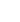15min:
THE RAMAN FREQUENCY SHIFTS RELATED TO THE THERMODYNAMIC QUANTITIES CLOSE TO THE PHASE I - PHASE II TRANSITION IN NH4Br.

H. YURTSEVEN, Department of Physics, Middle East Technical University, Ankara, 06531 - Turkey.

We establish here the modified Pippard relations by relating the specific heat Cp to the frequency shifts \frac1\left( \frac\partial\partial T \right)P and also the thermal expansivityp to the \frac1\left( \frac\partial\partial P \right)T for the(140 cm-1) Raman mode of NH4Br close to its phase I- phase II transition. From our linear plots of Cp vs. \frac1\left( \frac\partial\partial T \right)P andp vs.p to the \frac1\left( \frac\partial\partial P \right)T , we extract the values of the slope DP/dT, which are close to the experimental value of 28.2 bar/oC near the phase I-phase II transition in NH4Br.